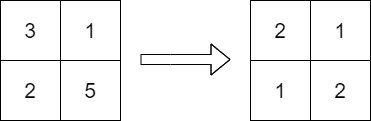##### Welcome to Subscribe On Youtube

Formatted question description: https://leetcode.ca/all/2371.html

# 2371. Minimize Maximum Value in a Grid

## Description

You are given an m x n integer matrix grid containing distinct positive integers.

You have to replace each integer in the matrix with a positive integer satisfying the following conditions:

• The relative order of every two elements that are in the same row or column should stay the same after the replacements.
• The maximum number in the matrix after the replacements should be as small as possible.

The relative order stays the same if for all pairs of elements in the original matrix such that grid[r1][c1] > grid[r2][c2] where either r1 == r2 or c1 == c2, then it must be true that grid[r1][c1] > grid[r2][c2] after the replacements.

For example, if grid = [[2, 4, 5], [7, 3, 9]] then a good replacement could be either grid = [[1, 2, 3], [2, 1, 4]] or grid = [[1, 2, 3], [3, 1, 4]].

Return the resulting matrix. If there are multiple answers, return any of them.

Example 1:Input: grid = [[3,1],[2,5]]
Output: [[2,1],[1,2]]
Explanation: The above diagram shows a valid replacement.
The maximum number in the matrix is 2. It can be shown that no smaller value can be obtained.


Example 2:

Input: grid = []
Output: []
Explanation: We replace the only number in the matrix with 1.


Constraints:

• m == grid.length
• n == grid[i].length
• 1 <= m, n <= 1000
• 1 <= m * n <= 105
• 1 <= grid[i][j] <= 109
• grid consists of distinct integers.

## Solutions

• class Solution {
public int[][] minScore(int[][] grid) {
int m = grid.length, n = grid.length;
List<int[]> nums = new ArrayList<>();
for (int i = 0; i < m; ++i) {
for (int j = 0; j < n; ++j) {
}
}
Collections.sort(nums, (a, b) -> a - b);
int[] rowMax = new int[m];
int[] colMax = new int[n];
int[][] ans = new int[m][n];
for (int[] num : nums) {
int i = num, j = num;
ans[i][j] = Math.max(rowMax[i], colMax[j]) + 1;
rowMax[i] = ans[i][j];
colMax[j] = ans[i][j];
}
return ans;
}
}

• class Solution {
public:
vector<vector<int>> minScore(vector<vector<int>>& grid) {
vector<tuple<int, int, int>> nums;
int m = grid.size(), n = grid.size();
for (int i = 0; i < m; ++i) {
for (int j = 0; j < n; ++j) {
nums.push_back({grid[i][j], i, j});
}
}
sort(nums.begin(), nums.end());
vector<int> rowMax(m);
vector<int> colMax(n);
vector<vector<int>> ans(m, vector<int>(n));
for (auto [_, i, j] : nums) {
ans[i][j] = max(rowMax[i], colMax[j]) + 1;
rowMax[i] = colMax[j] = ans[i][j];
}
return ans;
}
};

• class Solution:
def minScore(self, grid: List[List[int]]) -> List[List[int]]:
m, n = len(grid), len(grid)
nums = [(v, i, j) for i, row in enumerate(grid) for j, v in enumerate(row)]
nums.sort()
row_max =  * m
col_max =  * n
ans = [ * n for _ in range(m)]
for _, i, j in nums:
ans[i][j] = max(row_max[i], col_max[j]) + 1
row_max[i] = col_max[j] = ans[i][j]
return ans


• func minScore(grid [][]int) [][]int {
m, n := len(grid), len(grid)
nums := [][]int{}
for i, row := range grid {
for j, v := range row {
nums = append(nums, []int{v, i, j})
}
}
sort.Slice(nums, func(i, j int) bool { return nums[i] < nums[j] })
rowMax := make([]int, m)
colMax := make([]int, n)
ans := make([][]int, m)
for i := range ans {
ans[i] = make([]int, n)
}
for _, num := range nums {
i, j := num, num
ans[i][j] = max(rowMax[i], colMax[j]) + 1
rowMax[i] = ans[i][j]
colMax[j] = ans[i][j]
}
return ans
}

func max(a, b int) int {
if a > b {
return a
}
return b
}

• function minScore(grid: number[][]): number[][] {
const m = grid.length;
const n = grid.length;
const nums = [];
for (let i = 0; i < m; ++i) {
for (let j = 0; j < n; ++j) {
nums.push([grid[i][j], i, j]);
}
}
nums.sort((a, b) => a - b);
const rowMax = new Array(m).fill(0);
const colMax = new Array(n).fill(0);
const ans = Array.from({ length: m }, _ => new Array(n));
for (const [_, i, j] of nums) {
ans[i][j] = Math.max(rowMax[i], colMax[j]) + 1;
rowMax[i] = colMax[j] = ans[i][j];
}
return ans;
}